Catmull-Rom Splines Demystified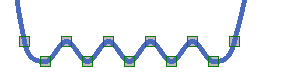Anthony Littlewood BSc

Brief:

The entire universe around us is built on fundamental mathematical constructs, these constructs must be replicated if any form of motion is to be possible inside a game, many beginners struggle with this and an even larger number of people have no idea of the significance of mathematics in computer graphics.

I wrote this article mainly for people who have an interest in the maths behind games, while there are many pieces of reference material available that can be applied to simulation, many of them are quite frankly terrifying. This Article is here to provide an easy introduction to the core mathematics that are used to run motion in a game. Note that I use the term motion here where many people would use the term physics or dynamics, I do this because I feel the term physics, is subjective and open to interpretation, it is my own feeling that referring instead to motion will keep things easier to understand, also a lot of people are immediately intimidated by the concept of the maths behind physics.

Prerequisites:

1. at least half a partially functioning brain

I also feel that other things would be useful but are not essential

1. some programming background
2. some mathematical skills
3. a calculator or alternatively a full partially functioning brain

I also have functioning examples of the code running, available feel free to look at these, experiment with them, and try to understand.

If this information is new to you you may get lost, I have tried to create a very gradual learning curve on the information to help, thank you for reading.

Introduction:
This is an article I wrote in an attempt to clarify and demystify spline curves. Trying to find references, that would allow me to actually understand how they worked I found very difficult. Many articles and books I have read present them in very abstract terms of mathematics that make translation to code challenging. The code that I found was convoluted, and didn’t actually explain any of what was going on. There were also very few “drag and drop libraries” available, considering how useful and inherently general Splines are, so I decided it was time to start writing.

Argh My Spline!:
Splines are a mathematical way of defining a path between a series of points, they have many uses in graphics and mathematics, common uses in games include generating dynamic level of detail meshes and defining cinematic camera paths.

generally speaking,cubic curves/splines are a natural progression from the parametric equations used in interpolation, and thus the formulas have a cross-over point.
Enter The “LERP”: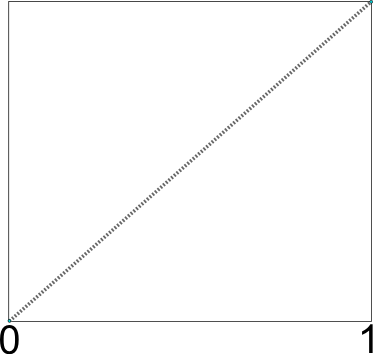the basic formula for basic linear interpolation is very simple: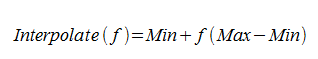Unfortunately, both the timing and the velocity produced by this formula are linear, this means the formula will not smooth the paths nor will it adjust the speed and acceleration of an object on the path. it will simply move at a constant velocity until it hits the end before stopping dead, this without smoothing it looks very inorganic and mechanical.

Fortunately we can make use of a smoothstep formula for smoothing the path:

Enter The Smoothstep: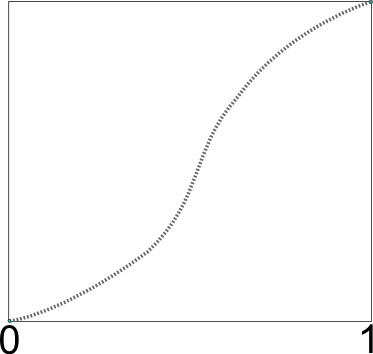The formula for smoothstep is: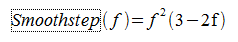Squaring the input value(also known as raising to the power of two) here is key, the higher the power the smoother the effect.

This function essentially gives us a curve, if we then feed this into the linear interpolation function we get much the same results as before but our output will accelerate up to the centre point and then slow down again as it moves away from the centre.

These curves look very nice but will only work between two points, if we wish to have a roller coaster following a string of points we cannot do it with this method.

Ruptured Spline !:
Splines are smooth/curved paths that are constructed from “control points”.

The one I am going to describe here is known as a Catmull-Rom spline, I personally like Catmull-Rom splines, they look nice and don’t give strange artifacts. They are also guaranteed to hit all of the control points provided(there is one exception which I will explain in a moment), this makes them fantastic for camera paths and animation.

The important point to remember about Catmull-Rom splines however is that while the first and last control points do have an effect on the final curve, they do not form part of the actual visual curve, only the centre points do: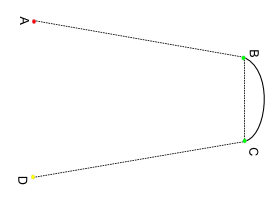In this diagram for example, the points A and D do not form part of the visible curve, if this is extended over a list of points, likewise the first and last points of the list would not be drawn

Formula Time:
Okay, so the formula itself: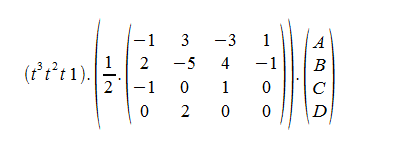Ta-daa, pretty right?

If you don’t have a background in mathematics, this looks scary at first, but there really isn’t anything overly difficult to it.

What Does It All Mean!?
Firstly those dots are just multiplications, throughout primary school, children are taught from a young age that the universally accepted symbol for multiplication is an “X”… unfortunately, all primary school mathematics teachers are liars.

Since the character X is so often used in algebra to label a variable, clearly identifying what is a multiply and what is an “X” becomes a problem, this is why the symbol is left out, as in “3 multiplied by A”  is actually written as “3A”.

A dot is specifically an indicator for a “dot-product” operation also known as a “scalar product”, meaning that we will end up with a single floating point number(also known as a “scalar”).

if you come from a programming background like myself however the symbol for basic multiplication is an asterisk “*”.

In this article I will show formulas with dots and working maths/code with asterisks.

Erm the ABCD?
These are variables simply representing our control points, I should point out here that the formula only needs to calculate in one dimension, this is because there is no difference in the implementation between the x, y or z axis so we might aswell just run everything again for each dimension instead of complicating the formula unnecessarily.

The one dimensional formula means that the control points are just numbers on a one dimensional line (or phase as some people call them). As I said above though, remember that the curve will only actually be drawn between B and C.

The ½ obviously just means we need to half our result to get what we want, this is just a simple part of the formula and since halving is so trivial we might as well ignore it for the moment and just half the result of everything once we have done the hard part.

The first bit: ( t cubed t squared t 1) is common across cubic functions: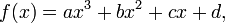The long shot of all this craziness is that it returns a floating point number. The a, b, c, d here are what I like to think of as connection points for us to plug equations into the core cubic function, these extra equations are where we find the differences between all the different cubic equations. this formula itself, however is basically just a common model or a base plate for all of them.

What Is That Large Terrifying Matrixy Bit:
Earlier I mentioned that the core cubic function is basically a baseplate for the other equations that hook into it, those extra functions are are grouped together and stacked within that matrix, they are called “Polynomials”.

What The Crap Is A Polynomial?:
wikipedia states the following:
“In mathematics, a polynomial is an expression of finite length constructed from variables (also known as indeterminates) and constants, using only the operations of addition, subtraction, multiplication, and non-negative integer exponents.”

Again, as with most things in mathematics, someone comes up with these things then gives them a terrifying name, and an even more terrifying description, for reasons only a mathematician can understand.

Thus I present my “alternative” name:
“Non-terrifying equation-y thing”™

And description:
A “non-terrifying equation-y thing”, is a small equation generally dropped into part of a larger equation, since there is usually a number of them stuck together inside a deceptively complex looking matrix, they look absolutely horrifying, but the actual numbers and mathematics in them is usually quite basic and for all the fluff, all they actually do is return a number.

The matrix above is a 4×4 matrix, in the format drawn above it is intended to be multiplied against the vector(or one dimensional matrix) of control points, each row of the matrix is  a “non-terrifying equation-y thing”, so all we have to do is take each “non-terrifying equation-y thing” and multiply it by the appropriate indexed number in our vector of control points.

OMG Pixels! :
To demonstrate what this formula actually creates I made a little application in Processing to draw a simple two dimensional Catmull-Rom spline: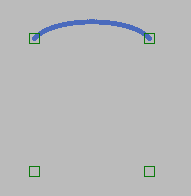As you can see, points A and D do not get drawn as part of the curve and just act as control points, also, to get the curve to draw as a solid line, after calculating the polynomials, I simply tween between 0.0 and 1.0, by a very small amount in a loop, and draw a small circle at each point along the way, the result is a fairly clean looking line.

It Needs More… “Splineyness”:
Extending to draw more control points is trivial, we can simply grab four points from the list of many, draw them as listed here, then iterate forwards by one, draw again, and keep going until we run out of points in the list.

I expanded on the application and made it able to to read points in from an xml file and draw them using the Catmull-Rom spline formula. I decided Inkscape was a good application for creating a demonstration shape, unfortunately I am not an artist so all I was inspired to create was a very bad looking skull type shape:

You can see the skull I made here on the right,
and the nice smooth one with the same input data being rendered by the Catmull-Rom formula on the left, I have included the application with this article, if you want to, feel free to open the xml and add some points.

The Actual Mathematics:

I felt that if I put this part in the middle it would probably make some people’s brains melt since it is tragically boring, it’s also rather pointless since the actual number work is done by the cpu anyway, so if you don’t have an interest in mathematics please feel free to skim over this…

Alternatively if you are insane or just want to better understand how the maths breaks down keep reading and good luck.

(Remember: When multiplying matrices like this, the index of one matrix needs to go across while the other needs to go down.)

(If it’s easier, think they both go across but the vector only has one element across so carries over to the next line down.)

Our first “Polynomial” (read: “non-terrifying equation-y thing”/ row from the matrix) is:
-1,3,-3,1

Okay, so firstly any number that isn’t negative is positive so let’s write that in just to make it clear:
-1,+3,-3,+1

okay, does it still look terrifying now?

If yes ask an eight year old what they think,

If no, well done, you have got over the hard bit, now it’s smooth sailing.

we have to multiply each control point (ABCD) by each of these numbers in turn, so:

-1*A, +3*B, -3*C, +1*D.

simple right? but, we can make it even simpler, since anything multiplied by 1 is itself we can stop writing the 1’s in, but we do need to keep the positive or negative.

-A, +3*B, -3*C, +D.

Now remember earlier when I mentioned that all a “Polynomial” (read: non-terrifying equation-y thing) does is return a number ? well you should be able to see how this works now, if we remove those commas, look what we end up with for the first polynomial:

result = -A + 3 * B – 3 * C + D

Remember that each of the letters is just a control point(or a variable), and we are only working in one dimension so all those letters really are is more numbers,( probably really nice ones), but the truth is, you don’t actually need to care, since that grunt work is going to be the computer’s job anyway.

Isn’t that just lovely?

Now if you take a quick look back at that matrix, it should be looking far less intimidating by now.

You should also see that there are still three polynomials to figure out, and we have already done the “difficult” one.

The second one is:

2, -5, 4, -1,

Let’s follow the same process as before, we should end up with:

+2, -5, +4, -1

+2A -5B +4C -1D

Again, we don’t need that one written in there and the starting “+” is pointless since we all know the first number is not negative:
2A – 5B + 4C – D

so we end up with:
result = 2*A – 5*B + 4*C – D

Medical Attention Period/Tea Break:
if you are still with me then feel free to wheel past the other two polynomials since they are  much of the same except comically simple.

Alternatively if your ears are bleeding at the moment but you have been otherwise enjoying learning about splines so much you couldn’t leave, you now have my permission to seek medical attention, or at least get a cup of tea then come back since the other polynomials as I said are really simple, and I will breeze through them just for the sake of completeness:

Intermission / A Brief Recess:
Okay, so you came back I see?, well thats good. Lets finish the rest of these Polynomials.

Polynomial C:
-1, 0, 1, 0
Does the matrix still look scary? nope? good.

-1, +0, +1, +0

-1*A +0*B +1*C +0*D

we can strip out any multiplies by 0 since they don’t actually do anything (yay!)
-1*A +1*C

result = -A + C;

Polynomial D <- (the really really really easy one):

0, 2, 0 ,0

+0,+2,+0,+0

+0*A, +2*B, +0*C,+0*D
we can scrap almost all of this:

result = 2*B;

Okay so we finally got here, if you didn’t understand before but now you do, well done, seriously, well done. see how foolish that formula looks now, the formula that was once so high and mighty with its shiny “Polynomials”. At this point you can feel free to scroll back up to it, and laugh maniacally at the silly formula and all it’s foolishness.

Okay, so we have our polynomials calculated, we have accounted for our control points, we have successfully mocked the formula, and we know what a cubic equation looks like, now how do we stick it all together again:

Sticking it all back together:
This is the really easy bit, we just need to feed the results from our Polynomials(read: non-terrifying equation-y thing) into our standard cubic baseplate, you will notice, by the way that it is also a vector:

(t³, t², t  1)
You will also notice that it goes across instead of up, I will explain why in a moment.

You will also notice that this is supposed to be multiplied against something, namely our polynomials, we already have the results of all these so it’s easy, lets grab them from up there ^ where we figured them out:

PolyA = -A + 3 * B – 3 * C + D
PolyB = 2*A – 5*B + 4*C – D
PolyC = -A +C;
PolyD = 2*B;

Okay, now we have them we chuck them together and stack them up into a vertical vector like this:

Since our cubic base vector is horizontal, we can just multiply them against one another:

(t³, t², t  1)  .

Writing that out so we can actually do something with it we get this:

PolyA * t³, PolyB*t², PolyC*t, PolyD*1

Again as before let’s add our signs in:
+PolyA * t³, +PolyB*t², +PolyC*t, +PolyD*1

And strip what we don’t need to say:
PolyA * t³, +PolyB*t², +PolyC*t, +PolyD

Now, if we just remove the commas we get our final formula:
PolyA * t³ + PolyB * t² + PolyC * t + PolyD

This is where we can drop the divide by 2 in, we could have done it sooner, and applied it to all the Polynomials separately, but there doesn’t seem to be much point since the net result is the same either way and we can save some legwork:

(PolyA * t³ + PolyB * t² + PolyC * t + PolyD) / 2;

There we go, easy. So to get our actual point we just have to feed it a value for “t” and then draw our result:

Behold : My Mighty Polynomials!
CatmulRom(t) = (PolyA * t³ + PolyB * t² + PolyC * t + PolyD) / 2;

the great thing about this is if we were to separate the polynomials matrix in code, we could avoid having to recalculate them every time we request a new point on the curve, since they don’t need to be recalculated unless the control points move or change.

If we go through the process twice we can apply it to a second dimension and actually render the curve we want and finally we can get to see something interesting for all our hard work, furthermore, going through the process a third time, we can draw a 3 dimensional curve.

Conclusion (Oh Thank God):
Well if you followed along all the way through(without lapsing into a coma) then thank you and well done, we have come a long way together, and hopefully you can now do Catmull-Rom splines, if there is anything you want to ask me, feel free, if you want to learn more there are links in the “Additional References” I can strongly recommend.

Thanks again and Kindest Regards -Anthony Littlewood BSc (hons)
NGangst@goowy.com
antsportfolio.co.uk